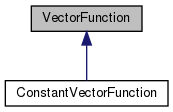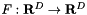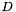Chombo + EB  3.0
VectorFunction Class Referenceabstract

#include <VectorFunction.H>

Inheritance diagram for VectorFunction:[legend]

## Public Member Functions

VectorFunction (bool a_homogeneous, bool a_constant)

virtual ~VectorFunction ()
Destructor. More...

virtual RealVect operator() (const RealVect &a_x, Real a_t) const =0

virtual RealVect derivative (const IntVect &a_order, const RealVect &a_x, Real a_t) const

virtual bool hasDerivative (const IntVect &a_order) const

RealVect operator() (const RealVect &a_x) const

RealVect derivative (const IntVect &a_order, const RealVect &a_x) const

bool isHomogeneous () const
Returns true if this function is homogeneous, false otherwise. More...

bool isConstant () const
Returns true if this function is constant, false otherwise. More...

## Protected Attributes

bool m_isHomogeneous

bool m_isConstant

## Private Member Functions

VectorFunction ()

VectorFunction (const VectorFunction &)

VectorFunctionoperator= (const VectorFunction &)

## Detailed Description

This base class represents a vector function.

## ◆ VectorFunction() [1/3]

 VectorFunction::VectorFunction ( bool a_homogeneous, bool a_constant )

Base class constructor. Must be called by subclasses.

Parameters
 a_homogeneous This flag indicates whether the vector function is constant in space. a_constant This flag indicates whether the vector function is constant in time.

## ◆ ~VectorFunction()

 virtual VectorFunction::~VectorFunction ( )
virtual

Destructor.

## ◆ VectorFunction() [2/3]

 VectorFunction::VectorFunction ( )
private

## ◆ VectorFunction() [3/3]

 VectorFunction::VectorFunction ( const VectorFunction & )
private

## ◆ operator()() [1/2]

 virtual RealVect VectorFunction::operator() ( const RealVect & a_x, Real a_t ) const
pure virtual

Override this method to evaluate this function at the given point in space and time.

Parameters
 a_x A point in-dimensional space. a_t The time at which the function is evaluated.

Implemented in ConstantVectorFunction.

Referenced by operator()().

## ◆ derivative() [1/2]

 virtual RealVect VectorFunction::derivative ( const IntVect & a_order, const RealVect & a_x, Real a_t ) const
virtual

Override this method to evaluate the given partial derivative of the function at the given point in space and time.

Parameters
 a_order A multi-index identifying the order(s) of the partial derivative of the function to be evaluated. a_x A point in-dimensional space. a_t The time at which the derivative is to be evaluated.

Reimplemented in ConstantVectorFunction.

Referenced by derivative().

## ◆ hasDerivative()

 virtual bool VectorFunction::hasDerivative ( const IntVect & a_order ) const
virtual

Override this method to return true if the derivative of the requested order exists and is available, false if it is not. This must be implemented in a way that is consistent with the derivative method.

Parameters
 a_order A multi-index identifying the order(s) of the desired partial derivative of the function.

## ◆ operator()() [2/2]

 RealVect VectorFunction::operator() ( const RealVect & a_x ) const
inline

This evaluates the function at time 0.

Parameters
 a_x A point in-dimensional space.

References operator()().

## ◆ derivative() [2/2]

 RealVect VectorFunction::derivative ( const IntVect & a_order, const RealVect & a_x ) const
inline

This evaluates the given partial derivative of the function at time 0.

Parameters
 a_order A multi-index identifying the order(s) of the partial derivative of the function to be evaluated. a_x A point in-dimensional space.

References derivative().

## ◆ isHomogeneous()

 bool VectorFunction::isHomogeneous ( ) const
inline

Returns true if this function is homogeneous, false otherwise.

References m_isHomogeneous.

## ◆ isConstant()

 bool VectorFunction::isConstant ( ) const
inline

Returns true if this function is constant, false otherwise.

References m_isConstant.

## ◆ operator=()

 VectorFunction& VectorFunction::operator= ( const VectorFunction & )
private

## ◆ m_isHomogeneous

 bool VectorFunction::m_isHomogeneous
protected

Referenced by isHomogeneous().

## ◆ m_isConstant

 bool VectorFunction::m_isConstant
protected

Referenced by isConstant().

The documentation for this class was generated from the following file: